# Relaxation Oscillator using Op-amp

Published  February 6, 2020   0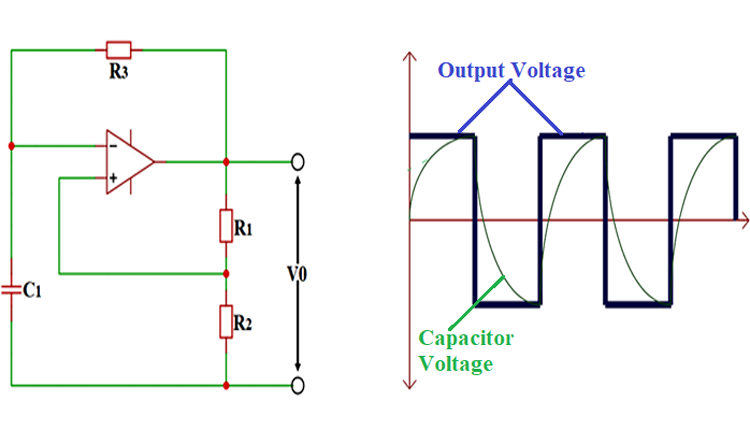Operational amplifier is an integral part of Electronics, and we previously learned about Op-amps in various op-amp based circuits and also built many oscillator circuits using op-amp and other electronics components.

Oscillator generally refers to the circuit which produces periodic and repetitive output like a sine wave or square wave. An oscillator can be a mechanical or electronic construction that produces oscillation depending on a few variables. Previously we learned about many popular oscillators like RC Phase shift oscillator, Colpitts oscillator, wein bridge oscillator, etc. Today we will learn about a Relaxation Oscillator.

A relaxation oscillator is the one which satisfies all the below conditions:

• It must provide a non-sinusoidal waveform (of either voltage or current parameter) at the output.
• It must provide a periodic signal or repetitive signal like Triangular, Square or Rectangular wave at the output.
• The circuit of a relaxation oscillator must be a nonlinear one. That means the design of the circuit must involve semiconductor devices like Transistor, MOSFET or OP-AMP.
• The circuit design must also involve an energy storing device like a Capacitor or Inductor which charges and discharges continuously to produce a cycle. The frequency or period of oscillation for such an oscillator depends on the time constant of their respective capacitive or inductive circuit.

### Working of a Relaxation Oscillator

For a better understanding of the Relaxation Oscillator, let us first look into the working of a simple mechanism shown below.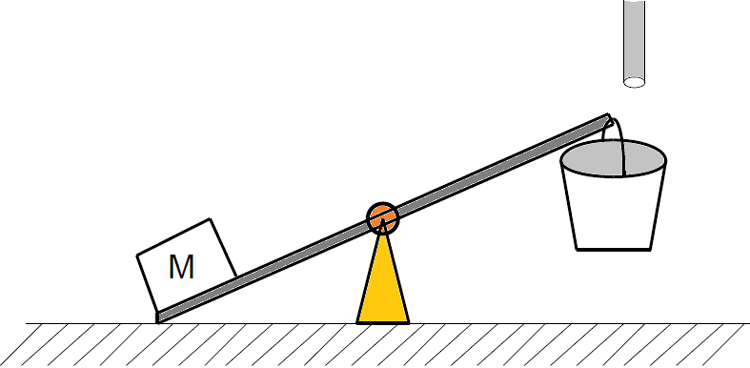The mechanism shown here is a seesaw that everyone probably experienced in their life. Plank moves back and forth depending on the gravitational force experienced by the masses on both ends. In simple terms, the seesaw is a comparator of ‘Mass’ and it compares the mass of objects placed on both the ends of the plank. So whichever objects has higher mass gets leveled to the ground while the lower mass object is lifted to air.

In this seesaw setup, we will have a fixed mass ‘M’ on one end and an empty bucket on the other end as shown in the figure. At this initial state the mass ’M’ will be leveled to the ground and the bucket will be hanged in the air based on seesaw principle discussed above.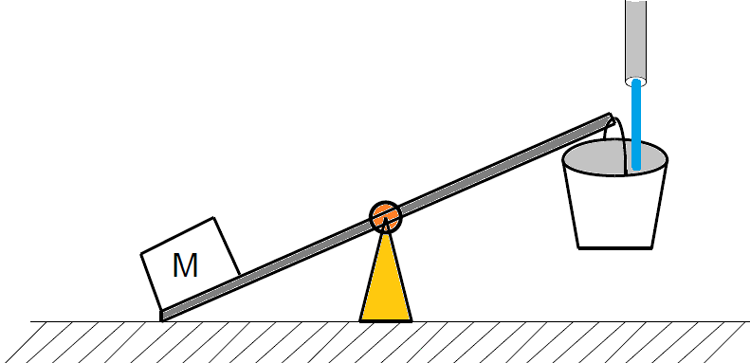Now, if turn on the tap placed above the empty bucket, then the water starts filling the empty bucket and thereby increasing the mass of the entire setup.

And once the bucket gets completely full, then the entire mass on the bucket side will be more than the fixed mass ’M’ placed on the other end. So the plank moves along the axis thereby airlifting the Mass ‘M’ and grounding the water bucket.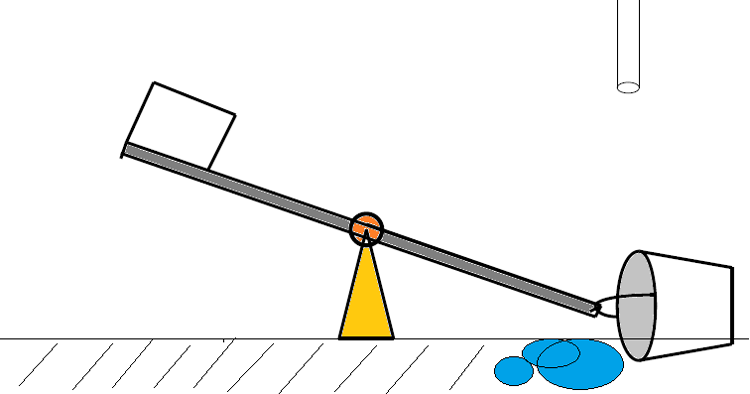Once the bucket hits the ground, the water filled in the bucket gets spilled over completely to the ground as shown in the figure. After the spillage, the total mass on the bucket side will again become less compared to the fixed mass ‘M’. So again the plank moves along the axis, thereby shifting the bucket to the air again for another filling.

This cycle of filling and spilling keeps on going up until the water source is present to fill the bucket. And because of this cycle, the plank moves along the axis with periodic intervals, thereby giving an oscillation output.

Now, if we compare the mechanical components with electrical components, then we have.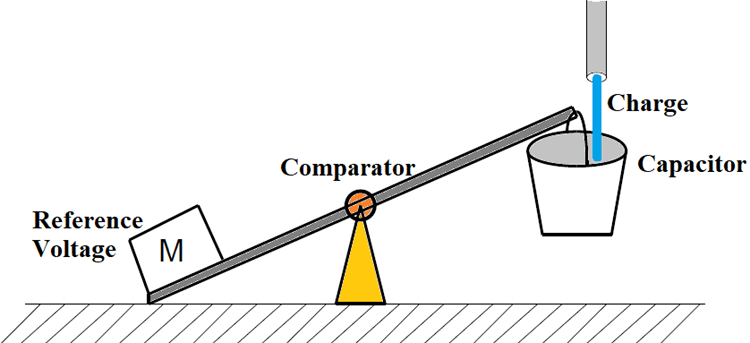• The bucket can be considered as an energy-storing device which is either a capacitor or an inductor.
• Seesaw is a comparator or an op-amp used for comparing the voltages of capacitor and reference.
• Reference voltage is taken for nominal comparison of capacitor value.
• The water flow here can be termed as an electric charge.

### Relaxation Oscillator Circuit

If we draw the equivalent electrical circuit for the above seesaw mechanism, we will get the Relaxation Oscillator Circuit as shown below: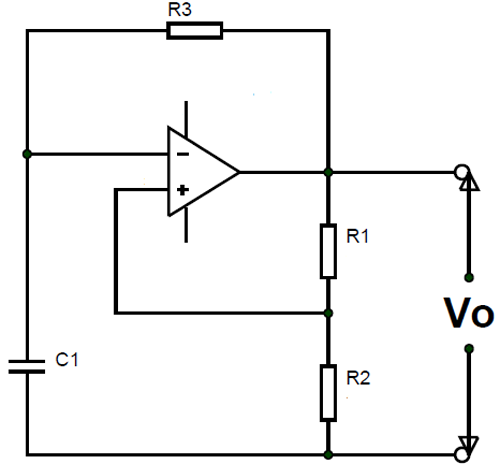The working of this Op-amp Relaxation Oscillator can be explained as follows:

• Once the tap is turned on, the water flows into a water bucket, thereby filling it slowly.
• After the water bucket is completely filled, the entire mass on the bucket side will be more than the fixed mass ’M’ placed on the other end. Once this happens, the plank shifts its positions to a more compromising place.
• After the water is completely spilled out, the total mass on the bucket side will again become less compared to the fixed mass ‘M’. So the shaft will move again to its initial position.
• Once again the bucket gets filled with water after the previous dispel and this cycle continues forever until there is water flowing from the tap.

If we draw the graph for the above case, it will look something like below: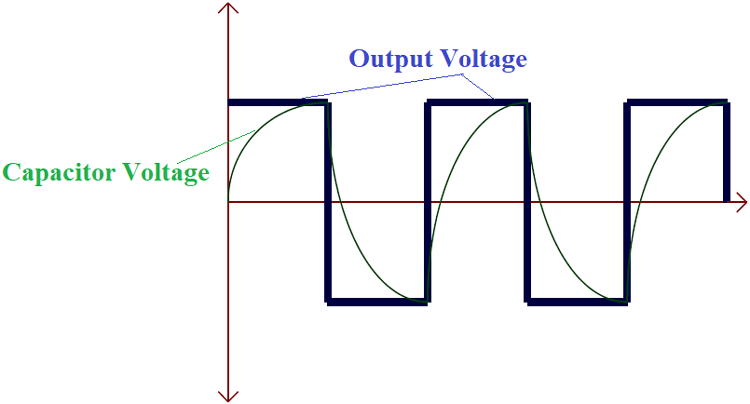Here,

• Initially, if we consider the output of the comparator is high, then during this time the capacitor will be charging. With the charging of the capacitor, its terminal voltage will gradually rise, which can be seen in the graph.
• Once the capacitor terminal voltage reaches the threshold, the comparator output will go from high to low as shown in the graph. And when the comparator output goes negative, the capacitor starts discharging to zero. After the capacitor completely discharges because of the presence of a negative output voltage, it again charges except in the opposite direction. As you can see in the graph because of the negative output voltage, the capacitor voltage also rises in a negative direction.
• Once the capacitor charges to the maximum in a negative direction, the comparator switches output from negative to positive. Once the output switches to a positive cycle, the capacitor discharges in the negative path and builds up charges in the positive path as shown in the graph.
• So the cycle of capacitor charge and discharge in positive and negative paths trigger the comparator produces a square wave signal at the output which is shown above.

### Frequency of Relaxation Oscillator

Obviously the frequency of oscillation depends on the time constant of C1 and R3 in the circuit. Higher values of C1 and R3 will lead to longer charge and discharge rates, thus producing lower frequency oscillations. Similarly, smaller values will produce higher frequency oscillations.

Here R1 and R2 also play a critical role in determining the frequency of the output waveform. This is because they control the voltage thresholds that the C1 needs to charge up to. For example, if the threshold set to 5V, then C1 only needs to charge and discharge up to 5V and -5V respectively. On the other hand, if the threshold is set to 10V, then C1 is needed to charge and discharge to 10V and -10V.

So the Relaxation Oscillator Frequency Formula will be:

`f = 1 / 2 x R3 x C1 x ln (1 + k / 1 - k)`
`Here, K = R2 / R1 + R2`

If the resistors R1 and R2 are equal to each other, then

`f = 1 / 2.2 x R3 x C1`

### Application of Relaxation Oscillator

Relaxation Oscillator can be used in:

• Signal generators
• Counters
• Memory circuits
• Voltage control oscillators
• Fun circuits
• Oscillators
• Multi-vibrators.